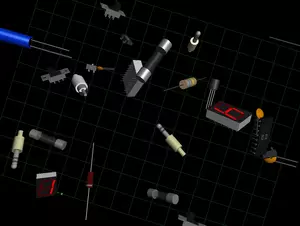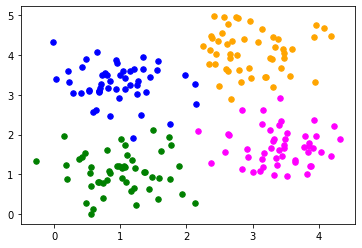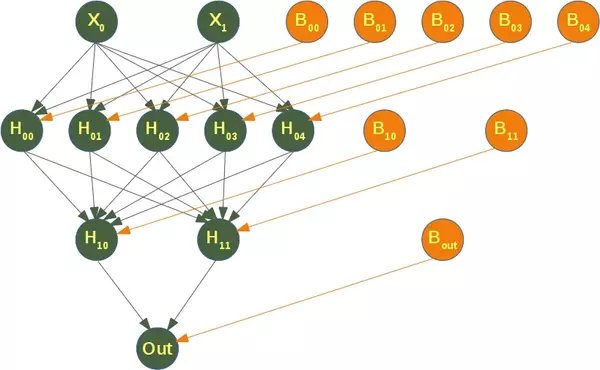# 22. Neural Networks with Scikit

## Introduction

In the previous chapters of our tutorial, we manually created Neural Networks. This was necessary to get a deep understanding of how Neural networks can be implemented. This understanding is very useful to use the classifiers provided by the sklearn module of Python. In this chapter we will use the multilayer perceptron classifier MLPClassifier contained in sklearn.neural_network

We will use again the Iris dataset, which we had used already multiple times in our Machine Learning tutorial with Python, to introduce this classifier.Live Python training

Enjoying this page? We offer live Python training courses covering the content of this site.

Enrol here

## MLPClassifier classifier

We will continue with examples using the multilayer perceptron (MLP). The multilayer perceptron (MLP) is a feedforward artificial neural network model that maps sets of input data onto a set of appropriate outputs. An MLP consists of multiple layers and each layer is fully connected to the following one. The nodes of the layers are neurons using nonlinear activation functions, except for the nodes of the input layer. There can be one or more non-linear hidden layers between the input and the output layer.

### Multilabel Example

import matplotlib.pyplot as plt
from sklearn.datasets import make_blobs

n_samples = 200
blob_centers = ([1, 1], [3, 4], [1, 3.3], [3.5, 1.8])
data, labels = make_blobs(n_samples=n_samples,
centers=blob_centers,
cluster_std=0.5,
random_state=0)

colours = ('green', 'orange', "blue", "magenta")
fig, ax = plt.subplots()

for n_class in range(len(blob_centers)):
ax.scatter(data[labels==n_class][:, 0],
data[labels==n_class][:, 1],
c=colours[n_class],
s=30,
label=str(n_class))from sklearn.model_selection import train_test_split
datasets = train_test_split(data,
labels,
test_size=0.2)

train_data, test_data, train_labels, test_labels = datasets


We will create now a MLPClassifier.

A few notes on the used parameters:

• hidden_layer_sizes: tuple, length = n_layers - 2, default=(100,)
The ith element represents the number of neurons in the ith hidden layer.
(6,) means one hidden layer with 6 neurons
• solver:
The weight optimization can be influenced with the solver parameter. Three solver modes are available

• 'lbfgs'
is an optimizer in the family of quasi-Newton methods.
• 'sgd'
• 'adam' refers to a stochastic gradient-based optimizer proposed by Kingma, Diederik, and Jimmy Ba

Without understanding in the details of the solvers, you should know the following: 'adam' works pretty well - both training time and validation score - on relatively large datasets, i.e. thousands of training samples or more. For small datasets, however, 'lbfgs' can converge faster and perform better.

• 'alpha'
This parameter can be used to control possible 'overfitting' and 'underfitting'. We will cover it in detail further down in this chapter.

from sklearn.neural_network import MLPClassifier

clf = MLPClassifier(solver='lbfgs',
alpha=1e-5,
hidden_layer_sizes=(6,),
random_state=1)

clf.fit(train_data, train_labels)


### OUTPUT:

/home/bernd/anaconda3/envs/python39/lib/python3.9/site-packages/sklearn/neural_network/_multilayer_perceptron.py:500: ConvergenceWarning: lbfgs failed to converge (status=1):
STOP: TOTAL NO. of ITERATIONS REACHED LIMIT.

Increase the number of iterations (max_iter) or scale the data as shown in:
https://scikit-learn.org/stable/modules/preprocessing.html
self.n_iter_ = _check_optimize_result("lbfgs", opt_res, self.max_iter)
MLPClassifier(alpha=1e-05, hidden_layer_sizes=(6,), random_state=1,
solver='lbfgs')
clf.score(train_data, train_labels)


### OUTPUT:

0.99375
from sklearn.metrics import accuracy_score

predictions_train = clf.predict(train_data)
predictions_test = clf.predict(test_data)
train_score = accuracy_score(predictions_train, train_labels)
print("score on train data: ", train_score)
test_score = accuracy_score(predictions_test, test_labels)
print("score on test data: ", test_score)


### OUTPUT:

score on train data:  0.99375
score on test data:  0.925

predictions_train[:20]


### OUTPUT:

array([0, 1, 3, 2, 1, 2, 0, 2, 0, 2, 0, 0, 0, 0, 2, 3, 3, 0, 3, 1])

## Multi-layer Perceptron

from sklearn.neural_network import MLPClassifier
X = [[0., 0.], [0., 1.], [1., 0.], [1., 1.]]
y = [0, 0, 0, 1]
clf = MLPClassifier(solver='lbfgs', alpha=1e-5,
hidden_layer_sizes=(5, 2), random_state=1)

print(clf.fit(X, y))


### OUTPUT:

MLPClassifier(alpha=1e-05, hidden_layer_sizes=(5, 2), random_state=1,
solver='lbfgs')


The following diagram depicts the neural network, that we have trained for our classifier clf. We have two input nodes $X_0$ and $X_1$, called the input layer, and one output neuron 'Out'. We have two hidden layers the first one with the neurons $H_{00}$ ... $H_{04}$ and the second hidden layer consisting of $H_{10}$ and $H_{11}$. Each neuron of the hidden layers and the output neuron possesses a corresponding Bias, i.e. $B_{00}$ is the corresponding Bias to the neuron $H_{00}$, $B_{01}$ is the corresponding Bias to the neuron $H_{01}$ and so on.

Each neuron of the hidden layers receives the output from every neuron of the previous layers and transforms these values with a weighted linear summation

$$\sum_{i=0}^{n-1}w_ix_i = w_0x_0 + w_1x_1 + ... + w_{n-1}x_{n-1}$$ into an output value, where n is the number of neurons of the layer and $w_i$ corresponds to the ith component of the weight vector. The output layer receives the values from the last hidden layer. It also performs a linear summation, but a non-linear activation function

$$g(\cdot):R \rightarrow R$$ like the hyperbolic tan function will be applied to the summation result.The attribute coefs_ contains a list of weight matrices for every layer. The weight matrix at index i holds the weights between the layer i and layer i + 1.

print("weights between input and first hidden layer:")
print(clf.coefs_)
print("\nweights between first hidden and second hidden layer:")
print(clf.coefs_)


### OUTPUT:

weights between input and first hidden layer:
[[-0.14203691 -1.18304359 -0.85567518 -4.53250719 -0.60466275]
[-0.69781111 -3.5850093  -0.26436018 -4.39161248  0.06644423]]

weights between first hidden and second hidden layer:
[[ 0.29179638 -0.14155284]
[ 4.02666592 -0.61556475]
[-0.51677234  0.51479708]
[ 7.37215202 -0.31936965]
[ 0.32920668  0.64428109]]


The summation formula of the neuron H00 is defined by:

$$\sum_{i=0}^{n-1}w_ix_i = w_0x_0 + w_1x_1 + w_{B_{11}} * B_{11}$$

which can be written as

$$\sum_{i=0}^{n-1}w_ix_i = w_0x_0 + w_1x_1 + w_{B_{11}}$$

because $B_{11} = 1$.

We can get the values for $w_0$ and $w_1$ from clf.coefs_ like this:

$w_0 =$ clf.coefs_ and $w_1 =$ clf.coefs_

print("w0 = ", clf.coefs_)
print("w1 = ", clf.coefs_)


### OUTPUT:

w0 =  -0.14203691267827162
w1 =  -0.6978111149778682


The weight vector of $H_{00}$ can be accessed with

clf.coefs_[:,0]


### OUTPUT:

array([-0.14203691, -0.69781111])

We can generalize the above to access a neuron $H_{ij}$ in the following way:

for i in range(len(clf.coefs_)):
number_neurons_in_layer = clf.coefs_[i].shape
for j in range(number_neurons_in_layer):
weights = clf.coefs_[i][:,j]
print(i, j, weights, end=", ")
print()
print()


### OUTPUT:

0 0 [-0.14203691 -0.69781111],
0 1 [-1.18304359 -3.5850093 ],
0 2 [-0.85567518 -0.26436018],
0 3 [-4.53250719 -4.39161248],
0 4 [-0.60466275  0.06644423],

1 0 [ 0.29179638  4.02666592 -0.51677234  7.37215202  0.32920668],
1 1 [-0.14155284 -0.61556475  0.51479708 -0.31936965  0.64428109],

2 0 [-4.96774269 -0.86330397],



intercepts_ is a list of bias vectors, where the vector at index i represents the bias values added to layer i+1.

print("Bias values for first hidden layer:")
print(clf.intercepts_)
print("\nBias values for second hidden layer:")
print(clf.intercepts_)


### OUTPUT:

Bias values for first hidden layer:
[-0.14962269 -0.59232707 -0.5472481   7.02667699 -0.87510813]

Bias values for second hidden layer:
[-3.61417672 -0.76834882]


The main reason, why we train a classifier is to predict results for new samples. We can do this with the predict method. The method returns a predicted class for a sample, in our case a "0" or a "1" :

result = clf.predict([[0, 0], [0, 1],
[1, 0], [0, 1],
[1, 1], [2., 2.],
[1.3, 1.3], [2, 4.8]])


Instead of just looking at the class results, we can also use the predict_proba method to get the probability estimates.

prob_results = clf.predict_proba([[0, 0], [0, 1],
[1, 0], [0, 1],
[1, 1], [2., 2.],
[1.3, 1.3], [2, 4.8]])
print(prob_results)


### OUTPUT:

[[1.00000000e+000 5.25723951e-101]
[1.00000000e+000 3.71534882e-031]
[1.00000000e+000 6.47069178e-029]
[1.00000000e+000 3.71534882e-031]
[2.07145538e-004 9.99792854e-001]
[2.07145538e-004 9.99792854e-001]
[2.07145538e-004 9.99792854e-001]
[2.07145538e-004 9.99792854e-001]]


prob_results[i] gives us the probability for the class0, i.e. a "0" and results[i] the probabilty for a "1". i corresponds to the ith sample.

Live Python training

Enjoying this page? We offer live Python training courses covering the content of this site.

Upcoming online Courses

Enrol here

## Complete Iris Dataset Example

from sklearn.datasets import load_iris


# splitting into train and test datasets

from sklearn.model_selection import train_test_split
datasets = train_test_split(iris.data, iris.target,
test_size=0.2)

train_data, test_data, train_labels, test_labels = datasets

# scaling the data
from sklearn.preprocessing import StandardScaler
scaler = StandardScaler()

# we fit the train data
scaler.fit(train_data)

# scaling the train data
train_data = scaler.transform(train_data)
test_data = scaler.transform(test_data)

print(train_data[:3])


### OUTPUT:

[[ 1.46727204 -0.17567492  1.2239981   1.19955976]
[-0.7719383   0.74088987 -1.31717443 -1.27107823]
[ 0.40659346  0.74088987  0.9416456   1.45962692]]

# Training the Model
from sklearn.neural_network import MLPClassifier
# creating an classifier from the model:
mlp = MLPClassifier(hidden_layer_sizes=(10, 5), max_iter=1000)

# let's fit the training data to our model
mlp.fit(train_data, train_labels)


### OUTPUT:

/home/bernd/anaconda3/envs/python39/lib/python3.9/site-packages/sklearn/neural_network/_multilayer_perceptron.py:614: ConvergenceWarning: Stochastic Optimizer: Maximum iterations (1000) reached and the optimization hasn't converged yet.
warnings.warn(
MLPClassifier(hidden_layer_sizes=(10, 5), max_iter=1000)
from sklearn.metrics import accuracy_score

predictions_train = mlp.predict(train_data)
print(accuracy_score(predictions_train, train_labels))
predictions_test = mlp.predict(test_data)
print(accuracy_score(predictions_test, test_labels))


### OUTPUT:

0.9083333333333333
0.9

from sklearn.metrics import confusion_matrix

confusion_matrix(predictions_train, train_labels)


### OUTPUT:

array([[41,  0,  0],
[ 0, 29,  0],
[ 0, 11, 39]])
confusion_matrix(predictions_test, test_labels)


### OUTPUT:

array([[ 9,  0,  0],
[ 0,  7,  0],
[ 0,  3, 11]])
from sklearn.metrics import classification_report

print(classification_report(predictions_test, test_labels))


### OUTPUT:

              precision    recall  f1-score   support

0       1.00      1.00      1.00         9
1       0.70      1.00      0.82         7
2       1.00      0.79      0.88        14

accuracy                           0.90        30
macro avg       0.90      0.93      0.90        30
weighted avg       0.93      0.90      0.90        30



## MNIST Dataset

We have already used the MNIST dataset in the chapter Testing with MNIST of our tutorial. You will also find some explanations about this dataset.

We want to apply the MLPClassifier on the MNIST data. We can load in the data with pickle:

import pickle

with open("data/mnist/pickled_mnist.pkl", "br") as fh:

train_imgs = data
test_imgs = data
train_labels = data
test_labels = data
train_labels_one_hot = data
test_labels_one_hot = data

image_size = 28 # width and length
no_of_different_labels = 10 #  i.e. 0, 1, 2, 3, ..., 9
image_pixels = image_size * image_size


### OUTPUT:

---------------------------------------------------------------------------
IndexError                                Traceback (most recent call last)
<ipython-input-23-2a513e046363> in <module>
8 train_labels = data
9 test_labels = data
---> 10 train_labels_one_hot = data
11 test_labels_one_hot = data
12

IndexError: tuple index out of range
mlp = MLPClassifier(hidden_layer_sizes=(100, ),
max_iter=480, alpha=1e-4,
solver='sgd', verbose=10,
tol=1e-4, random_state=1,
learning_rate_init=.1)

train_labels = train_labels.reshape(train_labels.shape,)
print(train_imgs.shape, train_labels.shape)

mlp.fit(train_imgs, train_labels)
print("Training set score: %f" % mlp.score(train_imgs, train_labels))
print("Test set score: %f" % mlp.score(test_imgs, test_labels))

fig, axes = plt.subplots(4, 4)
# use global min / max to ensure all weights are shown on the same scale
vmin, vmax = mlp.coefs_.min(), mlp.coefs_.max()
for coef, ax in zip(mlp.coefs_.T, axes.ravel()):
ax.matshow(coef.reshape(28, 28), cmap=plt.cm.gray, vmin=.5 * vmin,
vmax=.5 * vmax)
ax.set_xticks(())
ax.set_yticks(())

plt.show()


Live Python training

Enjoying this page? We offer live Python training courses covering the content of this site.

Enrol here

## The Parameter alpha

A comparison of different values for regularization parameter ‘alpha’ on synthetic datasets. The plot shows that different alphas yield different decision functions.

Alpha is a parameter for regularization term, aka penalty term, that combats overfitting by constraining the size of the weights. Increasing alpha may fix high variance (a sign of overfitting) by encouraging smaller weights, resulting in a decision boundary plot that appears with lesser curvatures. Similarly, decreasing alpha may fix high bias (a sign of underfitting) by encouraging larger weights, potentially resulting in a more complicated decision boundary.

# Author: Issam H. Laradji
# code from: https://scikit-learn.org/stable/auto_examples/neural_networks/plot_mlp_alpha.html

import numpy as np
from matplotlib import pyplot as plt
from matplotlib.colors import ListedColormap
from sklearn.model_selection import train_test_split
from sklearn.preprocessing import StandardScaler
from sklearn.datasets import make_moons, make_circles, make_classification
from sklearn.neural_network import MLPClassifier
from sklearn.pipeline import make_pipeline

h = .02  # step size in the mesh

alphas = np.logspace(-1, 1, 5)

classifiers = []
names = []
for alpha in alphas:
classifiers.append(make_pipeline(
StandardScaler(),
MLPClassifier(
solver='lbfgs', alpha=alpha, random_state=1, max_iter=2000,
early_stopping=True, hidden_layer_sizes=[100, 100],
)
))
names.append(f"alpha {alpha:.2f}")

X, y = make_classification(n_features=2, n_redundant=0, n_informative=2,
random_state=0, n_clusters_per_class=1)
rng = np.random.RandomState(2)
X += 2 * rng.uniform(size=X.shape)
linearly_separable = (X, y)

datasets = [make_moons(noise=0.3, random_state=0),
make_circles(noise=0.2, factor=0.5, random_state=1),
linearly_separable]

figure = plt.figure(figsize=(17, 9))
i = 1
# iterate over datasets
for X, y in datasets:
# split into training and test part
X_train, X_test, y_train, y_test = train_test_split(X, y, test_size=.4)

x_min, x_max = X[:, 0].min() - .5, X[:, 0].max() + .5
y_min, y_max = X[:, 1].min() - .5, X[:, 1].max() + .5
xx, yy = np.meshgrid(np.arange(x_min, x_max, h),
np.arange(y_min, y_max, h))

# just plot the dataset first
cm = plt.cm.RdBu
cm_bright = ListedColormap(['#FF0000', '#0000FF'])
ax = plt.subplot(len(datasets), len(classifiers) + 1, i)
# Plot the training points
ax.scatter(X_train[:, 0], X_train[:, 1], c=y_train, cmap=cm_bright)
# and testing points
ax.scatter(X_test[:, 0], X_test[:, 1], c=y_test, cmap=cm_bright, alpha=0.6)
ax.set_xlim(xx.min(), xx.max())
ax.set_ylim(yy.min(), yy.max())
ax.set_xticks(())
ax.set_yticks(())
i += 1

# iterate over classifiers
for name, clf in zip(names, classifiers):
ax = plt.subplot(len(datasets), len(classifiers) + 1, i)
clf.fit(X_train, y_train)
score = clf.score(X_test, y_test)

# Plot the decision boundary. For that, we will assign a color to each
# point in the mesh [x_min, x_max] x [y_min, y_max].
if hasattr(clf, "decision_function"):
Z = clf.decision_function(np.c_[xx.ravel(), yy.ravel()])
else:
Z = clf.predict_proba(np.c_[xx.ravel(), yy.ravel()])[:, 1]

# Put the result into a color plot
Z = Z.reshape(xx.shape)
ax.contourf(xx, yy, Z, cmap=cm, alpha=.8)

# Plot also the training points
ax.scatter(X_train[:, 0], X_train[:, 1], c=y_train, cmap=cm_bright,
edgecolors='black', s=25)
# and testing points
ax.scatter(X_test[:, 0], X_test[:, 1], c=y_test, cmap=cm_bright,
alpha=0.6, edgecolors='black', s=25)

ax.set_xlim(xx.min(), xx.max())
ax.set_ylim(yy.min(), yy.max())
ax.set_xticks(())
ax.set_yticks(())
ax.set_title(name)
ax.text(xx.max() - .3, yy.min() + .3, ('%.2f' % score).lstrip('0'),
size=15, horizontalalignment='right')
i += 1

plt.show()


## Exercises

### Exercise 1

Classify the data in "strange_flowers.txt" with a k nearest neighbor classifier.

Live Python training

Enjoying this page? We offer live Python training courses covering the content of this site.

Enrol here

## Solutions

### Solution to Exercise 1

We use read_csv of the pandas module to read in the strange_flowers.txt file:

import pandas as pd

names=["red", "green", "blue", "size", "label"],
sep=" ")
dataset


The first four columns contain the data and the last column contains the labels:

data = dataset.drop('label', axis=1)
labels = dataset.label
X_train, X_test, y_train, y_test = train_test_split(data,
labels,
random_state=0,
test_size=0.2)


We have to scale the data now to reduce the biases between the data:

from sklearn.preprocessing import StandardScaler
scaler = StandardScaler()
X_train = scaler.fit_transform(X_train) #  transform
X_test = scaler.transform(X_test) #  transform

from sklearn.neural_network import MLPClassifier

mlp = MLPClassifier(hidden_layer_sizes=(100, ),
max_iter=480,
alpha=1e-4,
solver='sgd',
tol=1e-4,
random_state=1,
learning_rate_init=.1)

mlp.fit(X_train, y_train)
print("Training set score: %f" % mlp.score(X_train, y_train))
print("Test set score: %f" % mlp.score(X_test, y_test))


Live Python training

Enjoying this page? We offer live Python training courses covering the content of this site.

Upcoming online Courses

Enrol here